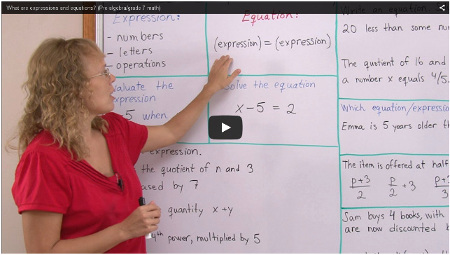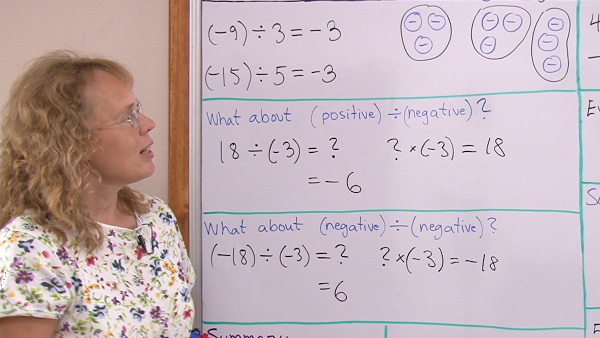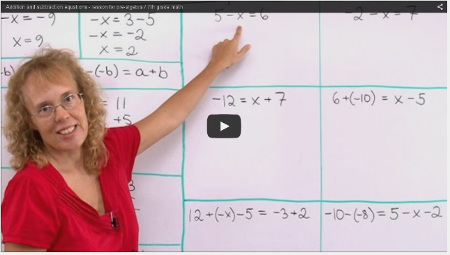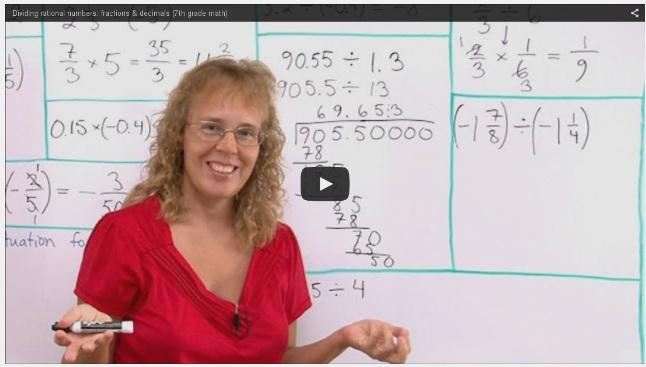^

# Free pre-algebra videos for middle school

This is a collection of free math videos for pre-algebra level (middle school), showing varied exercises for each topic. They match Math Mammoth Grade 7 curriculum but will also work no matter which curriculum you follow (in other words, the videos don't rely on you having Math Mammoth curriculum).

These videos are usable for students, teachers, and parents. You can use them...

• To learn these topics yourself (if you're a student for example, or an adult needing a refresher)
• As lesson plans for teaching these topics. Often, one video from below can be made into several lessons with students.

Please choose a pre-algebra topic from the list below to view a video tutorial for that topic.

## The language of algebra

The topics below provide an foundation to principles used in algebra (and pre-algebra).The order of operations

What are expressions & equations?

Working with expressions & equations

The commutative & associative properties

(The distributive property — simple version, for grade 6)

The distributive property — regular version, for grade 7 or pre-algebra

## Growing patterns

I have not made video lessons for growing patterns, but you can use this Youtube video for starters.

The other two I list below give a very mechanical way of approaching these types of pattern problems, where you don't have to think so much — but the method explained works always, so it can be helpful, especially if such a problem is expected to be on a standardized test:

However, my goal in putting the growing pattern problems in Math Mammoth Grade 7 is NOT to teach students a mechanical way, but just give them some exposure to thinking of different ways to SEE the pattern, and then try to express it using symbols. So there is no need to learn the mechanical way.

## Working with expressions

Simplifying and writing expressions 1: length and perimeter

Simplifying and writing expressions 2: area

Factoring linear expressions: using the distributive property backwards

## IntegersIntroduction to integers - grades 6-7

Subtraction of integers - grades 7-9

Multiplication of integers - grades 7-9

Division of integers - grades 7-9

Lessons on integers: solved examples - grades 7-9## The simplest equations

Solving equations - beginner lesson - examples with a pan balance

Multiplication and division equations

Writing simple equations for word problems

## Rational numbersWhat are rational numbers?

Add & subtract negative fractions and decimals

Multiply negative fractions and decimals

Divide rational numbers

Many operations with rationals, plus complex fractions

Unit rate word problem

Convert fractions to decimals

Simple equations involving decimals

Simple equations involving fractions

Simple equations involving percentages

## Linear equations

Two-step equations

Variable on both sides

Equations involving parentheses

Equations with fractions

How to use the formula for distance, rate (speed), and time to solve problems

Writing equations for word problems

Inequalities

Graphing equations

Introduction to slope

## Ratios and proportions

Solving proportions

Proportion word problems

Unit rates and more about proportions

How to find the unit rate, including with fractions

Proportional relationship or not?

Scaling figures and the scale ratio

Floor plans and scale ratio

Maps

## Percent

Basic percent word problems

Simple interest

Percentage of change

Percentage difference (relative difference)

Percent of increase/decrease: write an equation to solve for the original price

## Geometry

These lessons belong to Math Mammoth Grade 7 / pre-algebra course. Other pre-algebra courses may cover different geometry topics.

Unknown angle problems

Angles in a triangle

Drawing geometric shapes with given conditions

Drawing triangles with given conditions

Pi and the circumference of a circle

How to calculate the area of a circle, plus simple proof for the formula

## The Pythagorean Theorem

Square roots

Equations that require taking a square root

The Pythagorean Theorem

Applications of the Pythagorean Theorem 1: area of an isosceles triangle

Applications of the Pythagorean Theorem 2: diagonal of a square

Applications of the Pythagorean Theorem 3: volume of a triangular prism

Applications of the Pythagorean Theorem 4: perimeter of a trapezoid

## Probability and statistics

Introduction to probability

Probability of compound events: list the outcomes, draw a tree diagram

Practice problems for probability of compound events:

Data Analysis: Histogram

Data Analysis: Boxplot

Random sampling

Using random sampling

Comparing two populations

Back to all videos index

WAIT!

Receive my monthly collection of math tips & resources directly in your inbox — and get a FREE Math Mammoth book!You can unsubscribe at any time.

### Math Mammoth Tour

Confused about the different options? Take a virtual email tour around Math Mammoth! You'll receive:

An initial email to download your GIFT of over 400 free worksheets and sample pages from my books. Six other "TOURSTOP" emails that explain the important things and commonly asked questions concerning Math Mammoth curriculum. (Find out the differences between all these different-colored series!)

This way, you'll have time to digest the information over one or two weeks, plus an opportunity to ask me personally about the curriculum.
A monthly collection of math teaching tips & Math Mammoth updates (unsubscribe any time)### "Mini" Math Teaching Course

This is a little "virtual" 2-week course, where you will receive emails on important topics on teaching math, including:

- How to help a student who is behind
- Troubles with word problems
- Teaching multiplication tables
- Why fractions are so difficult
- The value of mistakes
- Should you use timed tests
- And more!

A GIFT of over 400 free worksheets and sample pages from my books right in the very beginning.A monthly collection of math teaching tips & Math Mammoth updates (unsubscribe any time)
Enter your email to receive math teaching tips, resources, Math Mammoth news & sales, humor, and more! I tend to send out these tips about once monthly, near the beginning of the month, but occasionally you may hear from me twice per month (and sometimes less often).• A GIFT of over 400 free worksheets and sample pages from my books.